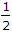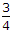# Civil Engineering - Strength of Materials - Discussion

### Discussion :: Strength of Materials - Section 3 (Q.No.13)

13.

The maximum stress intensity due to a suddenly applied load is x-times the stress intensity produced by the load of the same magnitude applied gradually. The value of x is

 [A]. 1 [B]. 2 [C]. 3 [D].[E].Explanation:

No answer description available for this question.

 Bikash Kabiraj said: (May 18, 2017) We know, that suddenly load is 2 times of gradually load.

 Rushi said: (Feb 17, 2019) Gradually applied load is given as σ = (F/A) where, F is the gradually applied load. here, work done is given as (F δL)/2. strain energy stored = (σ2 / 2E) AL. Work done is equal to the strain energy stored. (F δL) / 2 = (σ2 / 2E) AL so, σ = (F/A) --> (1) Suddenly applied load is given as σ = (2F/A), here work done = (F δL). (F δL) = (σ2 / 2E) AL. Therefore, σ = (2F/A) --> (2) From (1) and (2), it can be concluded that; Hence, suddenly applied load is twice the gradually applied load.